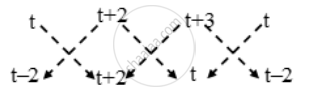# Prove that the area of a triangle with vertices (t, t −2), (t + 2, t + 2) and (t + 3, t) is independent of t. - Mathematics

Sum

Prove that the area of a triangle with vertices (t, t −2), (t + 2, t + 2) and (t + 3, t) is independent of t.

#### Solution 1

Let A(t, t − 2), B(t + 2, t + 2) and C(t + 2, t) be the vertices of the given triangle.

We know that the area of the triangle having vertices (x1, y1), (x2, y2) and (x3, y3) is

1/2|x_1(y_2-y_3)+x^2(y_3-y_1)+x_3(y_1-y_2)||

Area of ABC =  |1/2[x_1(y_2-y_3)+x^2(y_3-y_1)+x_3(y_1-y_2)||

=|1/2[t(t+2−t)+(t+2)(t−t+2)+(t+3)(t−2−t−2)]|

|1/2(2t+2t+4−4t−12)|

|-4|

=4 square units

Hence, the area of the triangle with given vertices is independent of t.

#### Solution 2therefore

rArr

rArr

rArr

⇒ Area of ∆ABC = 4 sq. units

Hence, Area of ∆ABC is independent of t.

Concept: Area of a Triangle
Is there an error in this question or solution?
2015-2016 (March) Delhi Set 1
Share